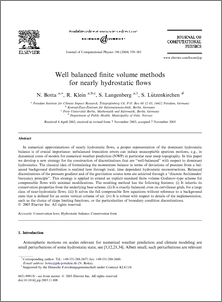Repository: Freie Universität Berlin, Math Department

# Well Balanced Finite Volume Methods for Nearly Hydrostatic Flows

Botta, N. and Klein, R. and Langenberg, S. and Lützenkirchen, S. (2004) Well Balanced Finite Volume Methods for Nearly Hydrostatic Flows. Journal of Computational Physics, 196 (2). pp. 539-565.Preview

1MB

## Abstract

In numerical approximations of nearly hydrostatic flows, a proper representation of the dominant hydrostatic balance is of crucial importance: unbalanced truncation errors can induce unacceptable spurious motions, e.g., in dynamical cores of models for numerical weather prediction (NWP) in particular near steep topography. In this paper we develop a new strategy for the construction of discretizations that are “well-balanced” with respect to dominant hydrostatics. The classical idea of formulating the momentum balance in terms of deviations of pressure from a balanced background distribution is realized here through local, time dependent hydrostatic reconstructions. Balanced discretizations of the pressure gradient and of the gravitation source term are achieved through a “discrete Archimedes' buoyancy principle”. This strategy is applied to extend an explicit standard finite volume Godunov-type scheme for compressible flows with minimal modifications. The resulting method has the following features: (i) It inherits its conservation properties from the underlying base scheme. (ii) It is exactly balanced, even on curvilinear grids, for a large class of near-hydrostatic flows. (iii) It solves the full compressible flow equations without reference to a background state that is defined for an entire vertical column of air. (iv) It is robust with respect to details of the implementation, such as the choice of slope limiting functions, or the particularities of boundary condition discretizations.

Item Type: Article Mathematical and Computer Sciences > Mathematics > Applied Mathematics Department of Mathematics and Computer Science > Institute of Mathematics > Geophysical Fluid Dynamics Group 921 Ulrike Eickers 29 Jul 2010 11:53 03 Mar 2017 14:40

Repository Staff Only: item control page